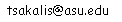EEE 582
Linear Systems
Fall 2017
TTh 4:30-5:45, ECG236

Instructor: Kostas Tsakalis, GWC 358, 965-1467,Textbook: Chen, Linear System Theory and Design, Oxford

Course Outline:

• Linear algebra review; Least-squares problems, singular value decomposition
• State-space concepts; description of dynamical systems, basic properties
• State Transition Matrix
• Stability
• Controllability-Observability
• Realizability, Minimal Realizations
• Canonical Forms
• Pole-Placement design of controllers and observers

Grading: HW 15%, 2 Midterms 50% total (drop worst score), Final 35%
Absolute Grading Scale: A > 85 > B > 70 > C > 50 > ... E
(Cut-off points may decrease depending on the final grade distribution.)

ALL TESTS ARE CLOSED-BOOKS/NOTES.  One sheet of formulae allowed.

Final Exam: (ASU SCHEDULE)

HW Assignments, Notes

HW#1, Due  9/7, Problems from the linked file EEE582 Homework Problems

EEE 582, Test 1, 9/14. Material: HW#1
Basic Definitions, System Models, Linearization, Continuous/Discrete-time systems.

HW#2, Due  9/21, Problems from the linked file EEE582 Homework Problems

EEE 582, Test 2, 9/28. Material: HW#2
Linear Algebra review, Jordan forms, SVD

HW#3 Due 10/5, Problems from the linked file EEE582 Homework Problems

EEE 582, Test 3, 10/12. Material: HW#3
Matrix exponential, Solution of State equations, Stability

HW#4  Due  10/26, Problems from the linked file EEE582 Homework Problems

EEE 582, Test 4, 11/2. Material: HW#4
Controllability, Observability

HW#5  Due 11/9, Problems from the linked file EEE582 Homework Problems

EEE 582, Test 5, 11/16. Material: HW#5
Realization Theory, Canonical forms (Kalman, Observable, Controllable, Balanced)

HW#6  Due  12/27, Problems from the linked file EEE582 Homework Problems

State Feedback , State Estimation, Observers, Output Feedback, Separation Principle

HW # Minimal Realization using Matlab
Consider the 2 x 2 6th order system defined by appending the tranfer functions of each input to each output:
H_11(s) = 1/(s+1), H_12(s) =  1/(s+1)+1/(s+2), H_21(s) = 1/(s+2), H_22(s) = 1/[(s+1)(s+2)]
1. Use the Kalman canonical decomposition theorem (see notes) to compute a minimal realization of this system
2. Use balanced truncation to compute reduced order models of orders 2 and 3 and use Bode plots/step responses to analyze the reduction error.